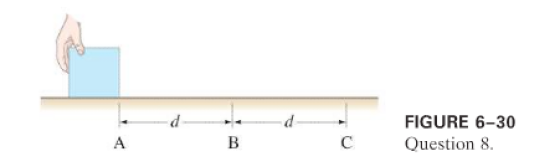×
Get Full Access to Physics: Principles With Applications - 6 Edition - Chapter 6 - Problem 8q
Get Full Access to Physics: Principles With Applications - 6 Edition - Chapter 6 - Problem 8q

×

# A hand exerts a constant horizontal force on a block thatISBN: 9780130606204 3

## Solution for problem 8Q Chapter 6

Physics: Principles with Applications | 6th Edition

• Textbook Solutions
• 2901 Step-by-step solutions solved by professors and subject experts
• Get 24/7 help from StudySoup virtual teaching assistantsPhysics: Principles with Applications | 6th Edition

4 5 1 264 Reviews
22
3
Problem 8Q

A hand exerts a constant horizontal force on a block that is free to slide on a frictionless surface (Fig.). The block starts from rest at point, and by the time it has traveled a distanceto pointit is traveling with speed $$v_{B}$$. When the block has traveled another distanceto pointwill its speed be greater than, less than, or equal to $$2 v_{B}$$ ? Explain your reasoning.Equation Transcription:Text Transcription:

v_B

2_vB

Step-by-Step Solution:

Solution 8Q:

Step 1 of 2:-

Here we have to find whether the speed of the block will be less than, greater than or equal to.

A constant horizontal force was applied on the block.

The block travelled the distance “d”.

The work done is,.

Again the same force is there and the block travelled “d” distance again.

So, the work done is again.

Step 2 of 2

## Discover and learn what students are asking

Unlock Textbook Solution